## Introduction

Labyrinth seals are extensively used in aero-engines to control the leakage between regions with different pressures to prevent the ingestion of hot gas in the turbine disk cavities and for cooling operations (Chupp et al., 2006). They are comprised of rotating and stationary components. The rotating parts are usually equipped with a series of radial fins while the stationary elements have straight or stepped geometries. The flow is repeatedly forced to pass through small clearances generating kinetic energy that is dissipated in the inter-fin cavity. This process increases the resistance to flow compared to a smooth slot.

The first pioneering studies on seal flutter were conducted during the 60’ and 70’ by Alford (1964, 1971, 1975). His works discuss the cause and prevention of fatigue failures in seals. He identified the importance of the support side of the seal in preventing self-excited vibration. Ehrich (1968) introduced the importance of the knife-edge clearance on the seal stability, but his findings were restricted to the 0th nodal diameter. Ehrich (1968) proposed an analytical model and identified a stability parameter that included the effect of the clearance, the support side of the seal, and the geometry of the inter-fin cavity. The models described by Ehrich and Alford did not take into account the effect of the circumferential flow. Abbot (1981) found that a seal supported on the low-pressure side was unstable only if the mechanical frequency was lower than the acoustic frequency of the seal, and vice-versa. According to Abbot’s criterion, the mechanical to acoustic frequency ratio and the support side of the seal are the only two parameters that control the stability of the seal.

All the previous studies were based on experimental analysis, failure evidence, and often on bulk-flow analytical models. Nowadays, CFD analyses are commonplace both in industry and academia. Mare et al. (2010) conducted a parametric investigation on a multi-finned straight-through seal to investigate the influence of the frequency and the support location on the seal stability. They found that the aerodynamic damping depended mainly on the cavity shape, the mode-shape, and the unsteady pressure phase distribution. Mare et al. (2010) describe the application of a time-marching nonlinear Navier-Stokes solver to the stability analysis of an LP turbine labyrinth seal for two different configurations. The results are compared with Abbot’s stability criterion suggesting that complex seal configurations are not appropriate to be treated with simple stability criteria based on the comparison of the structural and acoustic frequencies. Recently, Miura and Sakai (2019) have compared CFD results with experimental data obtained in an in-vacuum rotating rig. The numerical results show reasonably good agreement with the measurements.

Recently, Corral and Vega proposed a new comprehensive model for seal flutter (Corral and Vega, 2018; Vega and Corral, 2018). The model provides an expression for the work-per-cycle involving two new and additional dimensionless parameters to define the stability map of the seal. All the results of the classical analytical models (Abbot, 1981; Ehrich, 1968) are recovered and conciliated. The results were also extended to stepped-seals Corral et al. (2020). Moreover, it has been identified that the tip labyrinth seal has an outstanding effect on the stability of shrouded turbine rotor blades Corral et al. (2019). The numerical results are compared with the prediction of the Corral and Vega (CV) model corroborating the stabilizing effect predicted analytically. Recently, a new formulation of the baseline Corral and Vega model (2018) accounting for non-isentropic perturbations has been proposed (Corral et al., 2021b). The model redefines the dimensionless parameters described in Corral and Vega (2018) and outlines a more general stability map of the seal. The high sensitivity of the seal stability to differential gapping and the role of the effective gaps and the carry-over coefficient has been addressed recently (Corral et al., 2021a).

This work presents a numerical validation of the CV model for labyrinth seal flutter, including non-isentropic perturbations. The paper is organized as follows. First, the analytical model and the test case are briefly introduced. Secondly, steady-state results are presented. Finally, the results of the numerical simulations are compared with the predictions of the analytical model. It is concluded that the numerical results present a high degree of matching with the predictions of the high-order analytical model in a broad range of operating conditions provided that the model is properly informed with the seal steady-state obtained from a RANS solver.

## Governing equations

The baseline CV model (Corral and Vega, 2018) solves the linearised integral form of the mass-conservation equation of the inter-fin cavity and the differential form of the momentum equation in the circumferential direction. The circumferential unsteady variations of the flow are retained in the model but the mean flowfield is deemed spatially constant for a given circumferential position. The unsteady perturbations due to the seal vibration are assumed isentropic and varying only circumferentially. The isentropic condition is replaced by the energy equation of the inter-fin cavity in the higher-order version of the model (Corral et al., 2021b). The flow through the seal fin is deemed quasi-stationary since the thickness of the fin tip is much smaller than the characteristic size of the seal. Moreover, the process is assumed to be adiabatic and the work associated with the seal rotation in the inter-fin cavity is neglected. The seal vibration is introduced imposing that the spatio-temporal variations of the gaps, H, and the volume of the seal have the form of travelling-waves. Finally, the linearised equations are solved seeking solutions in the form of travelling waves as well.

The dimensionless out-of-phase component of the unsteady pressure of the cavity of the higher-order model is

##### (1)
p^outofphase=Ω¯e~heff+11St2/1~+Ω¯211St22

where the dimensionless pressure is defined as

##### (2)
p^=1εpcpc,swithε=LΔθHh

and where pc,s is the mean static pressure in the inter-fin seal cavity, pc the unsteady perturbation, and ε a small dimensionless parameter that is a function of the seal length, L, the gap, H, the angular vibration amplitude, Δθ, and h, a involved function of the seal pressure ratio (see Figure 1 for a summary of the nomenclature).

#### Sketch of the labyrinth seal geometry.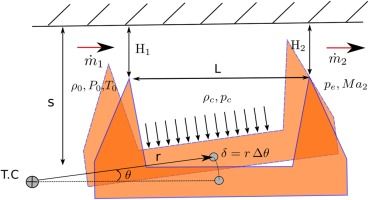Equation (1) reduces to a simple expression in the isentropic baseline model (Corral and Vega, 2018) since

##### (3)
Ω¯=ωpc,sVc,sm˙sa021hΩ^Ωh

where the auxiliary variable Ω^ varies smoothly between one and γ being the former the most common value in practical cases. Under the same conditions (relative high vibration frequencies) 1~1 as well. The reader is referred to Corral et al. (2021b) for further details. The mechanical to acoustic frequency ratio is defined as

##### (4)
St=ωRNDa0

In general, ω=ω(ND) and St can be expressed as a function of either the angular frequency, ω, or the nodal diameter, ND.

The dimensionless gap or torsion centre includes the effect of the pivot centre of the vibration mode-shape, r, the seal clearance, H, and the geometry of the seal (see Figure 1) and is defined as

##### (5)
e~=γrHsL

In the isentropic formulation of the problem (Corral and Vega, 2018), because of its simplicity, the parameters e~ and h appear always grouped as e~h which is very convenient. Nevertheless, in the higher-order formulation the parameter e~h is replaced by e~heff=e~hF(Ω) to account for non-isentropic perturbations. Moreover, the parameter e~heff is used to conceal other effects such as the slant of seal land (Corral et al., 2020) or the kinetic energy carried-over to the downstream fin (Corral et al., 2021a). The expression for h when the kinetic energy coefficient χ0 but the flow perturbations are considered isentropic is

##### (6)
h=h(πs)+J(πT/πs)(1χ)πsχ+(1χ)

where πs=P0/pc, πT=Po/pe and h and J are complex functions of the pressure ratio that can be found in the appendix of Corral and Vega (2018). The combined expression retaining simultaneously the kinetic energy carried-over downstream and the non-isentropic perturbations is too complex to be written here and does not add much to the discussion.

The dimensionless work-per-cycle, W~cyc, can be written as:

##### (7)
W~cyc=Wcycπpc,sδ2SL/|r|Hh=sign( r)p^outofphase

where δ=rΔθ is the seal torsion displacement in the land midpoint, and S=2πRL the surface of the seal land. The model accounts only for the work performed by the seal land since when the unsteady pressure is considered uniform within the inter-fin cavity the contribution of the fins cancels out unless the seal is slanted.

The dimensionless work-per-cycle depends on four non dimensional parameters

##### (8)
W~cyc=W~cyc(Ω¯,St,e~heff,h)

As described in Corral et al. (2021b) the dependence of the W~cyc on h is due to the new polytropic relationship included in the model. Moreover, e~heff introduces two limiting curves that bound the unstable region of the seal for each frequency, the boundary curves are obtained considering a seal with very low pressure ratio and a chocked seal. The physical interpretation of the parameters and their influence on the W~cyc are described in detail in Corral et al. (2021b) and Vega and Corral (2018).

## Numerical setup

A simplified geometry consisting of a straight-through labyrinth seal with a single inter-fin cavity has been constructed to assess the accuracy of the CV model. This model only accounts for the work exerted by the seal in the inter-fin cavity land which frequently is the dominant one. Therefore the actual shape of the adjacent cavities is irrelevant for this purpose. It is acknowledged that the neighbouring cavities, especially in or close to the acoustic resonances, can exhibit a large level of unsteadiness that eventually can influence the inter-fin cavity. Especial attention has been paid in this work to avoid such resonances to ease a clean comparison between the model and the CFD analyses. A schematic view of the model used in this study and the geometric details of the seal are shown respectively in Figure 1 and Table 1. Special care has been taken in sizing the upstream and downstream cavities. Both cavities have been designed to ensure a uniform pressure at the inlet and outlet of the seal and to avoid any eventual acoustic resonance in the frequency range of the simulations.

#### Physical geometric parameters of the seal inter-fin cavity.

Cavity radius (R)Nominal gap (H)Length (L)Height (s)
554 mm0.2 mm15.8 mm10.1 mm

The CV model has been already partially assessed on the tip-shroud of an LP turbine (Corral et al., 2019) using a well-validated frequency-domain linearized Navier-Stokes solver (Corral et al., 2003; Vega and Corral, 2016). In this work, a two-dimensional hybrid grid has been constructed in the meridional plane of the seal and extruded in the circumferential direction to form triangular prisms and hexahedra. A grid sensitivity study has been conducted by checking the convergence of the mass flow rate and the mean static pressure in the inter-fin cavity. Hereafter a model with approximately 350,000 points was chosen (see Figure 2). The standard kω turbulence model is used in this study and the mesh is fine enough to ensure y+1 in the whole domain. The model used in all the simulations is a sector of 10 with 10 layers in the circumferential direction. The seal geometry is considered axisymmetric, and phase-shifted boundary conditions were applied in the azimuthal interfaces to accommodate arbitrary ND in the 10-degree sector.

#### Outline of the computational domain around the seal (a), mesh detail around the fin tip clearance region (b), and azimuthal overview (c).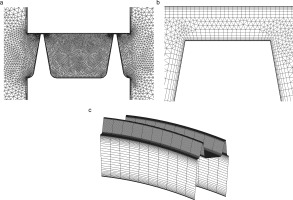### Mode-shape definition

The dimensionless work-per-cycle, W~cyc, of the seal flutter model (Corral et al., 2021b) is a function of four independent parameters namely, Ω¯, e~heff, heff and St. To ease the set up of the simulations independently changing each of these four parameters, a synthetic mode-shape generator has been implemented. The seal in the meridional plane is considered as a rigid body moving around a pivot point meanwhile in the azimuthal direction each section is shifted to a proper angle according to the selected nodal diameter. Figure 1 describes how the mode-shapes are defined in the meridional plane. The mode-shape displacements are applied on the seal wall nodes of the fluid mesh, and the inner points are moved using a Laplacian smoother. The displacements are small enough not to generate large distortions in the mesh that could jeopardize mesh quality. Moreover, this requirement is needed to ensure that the unsteady perturbations are linear. For a given mode, only the seal surface is moved, as shown in Figure 3a, while the rest of the solid walls are kept fixed. This method allows easily changing the pivot point, the ND, and the frequency for conceptual studies.

### Velocity streamlines for the nominal pressure ratio πT=2.0 colored by the static pressure contour (a), detail of the inter-fin cavity total pressure (b, bottom) and Mach number (b, top).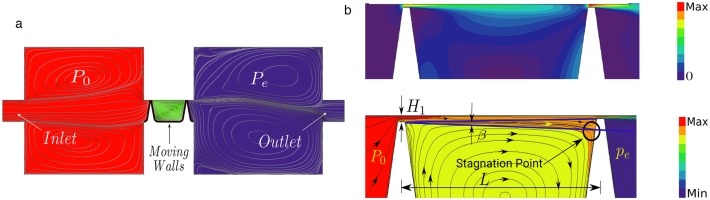## Results

The steady results obtained from the simulations are presented next. For all the simulations of this work, a non-rotating seal is assumed to reduce the uncertainties in comparing with the model. The high-pressure and low-pressure cavities are designed to act as plenum chambers. Both cavities are characterized by large low-Mach re-circulation zones. (see Figure 3b (top)). The streamlines displayed in the Figure 3b (bottom) show that the wall jet created in the first fin impinges onto the second one separating the inter-fin cavity into a thin wall-jet region and a large enclosed vortex. The wall-jet mixing increases with the distance dissipating his kinetic energy. The wall jet reaches the downstream clearance and part of its kinetic energy is recovered. The static pressure in the inter-fin cavity is nearly constant consistently with the CV model. The model has a built-in steady-state value however, the estimate of the static pressure within the cavity, the effective gaps, and the energy carried over to the downstream fin are obtained averaging the steady CFD solution.

It was observed that the discharge coefficient of the baseline case changes from the first to the second fin. To properly compare the numerical results with the model predictions we need to incorporate the effective gaps in the formulation. The discharge coefficient is defined as the ratio between the actual mass-flow, m˙, and the mass-flow in ideal conditions, m˙id. ratio Since the discharge coefficients of the first and second fin are different (Cd,1Cd,2) this automatically implies that even if the geometric gaps are identical, the effective clearances are not (H1,effH2,eff). The discharge coefficients estimated from the CFD are Cd,1=0.97 for the inlet fin and Cd,2=0.92 for outlet one whereas the kinetic energy carry-over coefficient is χ=0.2. The CV model includes the effective gaps by using the effective non-dimensional seal clearance parameter, e~eff which is a function of the ratio between the effective clearances η=H2,eff/H1,eff, which is η=0.83 in this particular case, and χ. For straight labyrinth seals with identical geometrical clearances, the effective gap ratio is defined as:

##### (9)
η=H2,effH1,eff=(1+ψ(1χ)χ)Cd2Cd1

where ψ=ψ(J,πs,χ) is a function of the pressure ratio across each fin and of the carry-over coefficient. (see Vega and Corral (2018); Corral et al. (2021a) for a detailed description of the model). The Reynolds number based on the gap of the seal is Reg=32,000 which is typical for this kind of configurations.

### Work-per-cycle: 0th nodal diameter

In this section, the prediction of the analytical model is compared against the numerical results obtained with a 2D linearized Navier-Stokes solver (Corral et al., 2003) for the 0th nodal diameter. The seal motion associated with this mode-shape does not create acoustic waves propagating in the azimuthal direction, and therefore there are no circumferential variations of the flow. The analytical expression of the work-per-cycle for the ND 0 is obtained from 1 for values of St. The stability map in Figure 4 compares the CFD simulations with the analytical model predictions. The non-dimensional work-per-cycle has been normalised by |1+e~effh|. The unsteady simulations are obtained for a pressure ratio of π=2.0, performing a frequency sweep up to a maximum dimensionless frequency of Ω~=3.6 for different torsion centers. The normalized work-per-cycle has been bounded to the range 1<W~/|1+e~effh|<1 to magnify the unstable zone.

### Dimensionless work-per-cycle contour plots of the 0th nodal diameter as a function of the dimensionless frequency, Ω~ and torsion centre, e~h′, for a two-fin straight seal operating at πT=2.0(h′=2). Left: CFD results. Right: analytical high-order model (Corral et al., 2021b). Black lines mark the stability limit (W~cyc=0) on the HPS.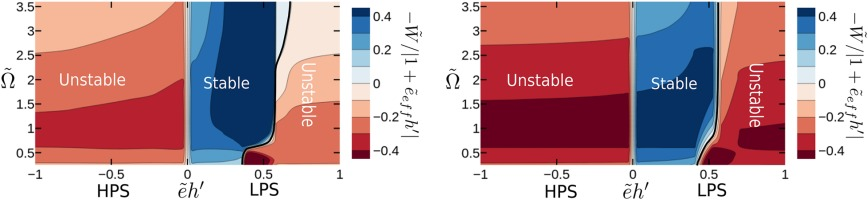The stability map shown in Figure 4 might seem weird at first glance, in fact, the seal is stable only in a narrow range of the nondimensional torsion centers (0<e~h<0.5) contrarily to what is predicted by the classical analytical models for seal flutter (see (Ehrich, 1968)). This stability pattern is a direct consequence of the effective gap ratio, η=0.83, due to the kinetic energy carried over to the downstream fin and the gap discharge coefficients, χ=0.2. As described in (Corral et al., 2021a), both effects significantly change the stability of the ND0, especially for high values of the nondimensional height of the seal, s¯=17.8, the stable region on the LPS suddenly decreases. In fact, the analytical expression of the stability criterion, which is obtained by imposing that the W~cyc=0:

##### (10)
ife~h<x>0thenη<x>2e~hs¯12e~h(1+s¯)+1

shows that for high values of the nondimensional height, s¯1, when the torsion center is located on the LPS and the effective gap ratio is η<1, the seal is stable only if e~h0. On the other hand, if the effective gap ratio is η=1, the seal is stable when the torsion centre is on the LPS, independently of the non-dimensional height, s¯. Figure 4 shows that the matching is good and sound overall, and the critical work-per-cycle is very well predicted. This proofs that most of the physics of the problem is retained by the analytical model.

The higher-order model (Corral et al., 2021b) predicts that for low-frequency of vibrations (Ω~1) the non-isentropic nature of the perturbations, which are isothermic, is responsible for the bending of the stability limit that otherwise would be a vertical line, this trend is retained by the CFD simulations. As a consequence of the normalization adopted, the maximum and minimum of the W~cyc occur in a small interval around e~h=0.5. It is important to highlight that around this value the torsion centre is physically far away from the seal mid-point in the simulations (r=+7L) and the seal motion no longer corresponds to a torsion mode. This means that during the vibration, the difference between the two clearances is small and the seal is not able to generate high unsteady pressure in the inter-fin cavity. Due to this, the physical work-per-cycle is very small compared with that obtained when |e~h|1. Moreover, one more effect that can be observed from the simulations when |r|1. Figure 5 shows the out-of-phase component of the unsteady pressure for a pressure ratio of π=2.0. It is clear that when the pivot point is far away from the inter-fin cavity (|e~h|1) the unsteady pressure in the inter-fin cavity is not uniform anymore (see Figure 5b), and its magnitude is close to that of the downstream cavity. In this context, even a small fraction of the unsteady pressure generated in the outer cavities could be transmitted through the clearances to the inter-fin cavity, leading to misleading results when comparing with the model. Therefore, it is not surprising that simulations over-predict the magnitude of the work-per-cycle of the stable zone when the seal is supported on the LPS. On the contrary, when the pivot point is nearby the inter-fin cavity (|e~h|1) the unsteady pressure generated in the inter-fin cavity (for the same amplitude of the seal displacement) is much higher and uniform in the inter-fin cavity (see Figure 5a).

### Influence of the inlet/outlet cavities: Out-of-phase component of the unsteady pressure for πT=2.0. (a): Modes with pivot points nearby the inter-fin cavity (|e~h′|≪1). (b): Modes with pivot points far away from the inter-fin cavity |e~h′|≫1.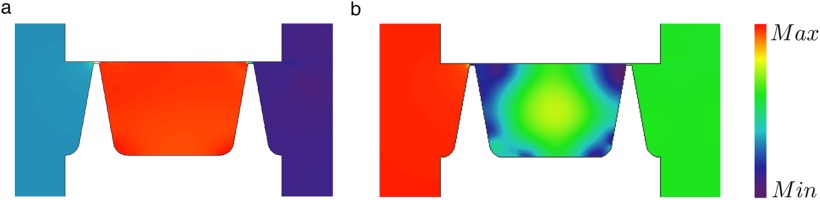Figure 6 shows the comparison of the CFD results with the analytical prediction for two selected torsion centers. The simulations have been obtained for a pressure ratio of πT=2 by changing the frequency of the seal while the torsion center was kept constant. The matching of the results for the LPS (Figure 6a) and the HPS (Figure 6b) torsion centers is good and the trend with the frequency is very well predicted.

### Nondimensional work-per-cycle for a two-fin straight seal for selected torsion centers and 0-th nodal diameter (πT=2). Solid Lines: CV model (Corral et al., 2021a). (a) e~h′=0.06(r/L=1) (b) e~h′=−0.23(r/L=−4) Symbols: Linearized Navier-Stokes Analyses.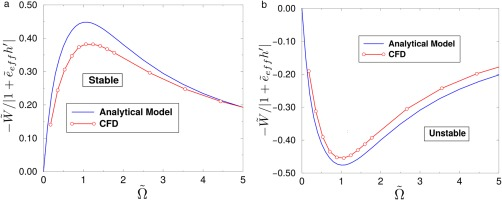### Work-per-cycle: Circumferential variations

The simulations of this section have been obtained by keeping the pressure ratio and the non-dimensional discharge time, Ω, constant. This method is used to reduce the risk of crossing the acoustic resonances of the adjacent cavities of the seal. As a consequence, the circumferential variations have been accounted for on the mechanical to acoustic frequency ratio, St, by changing the nodal diameter. In the high-order model, the critical reduced frequency, St, is obtained by imposing in Equation (7) that W~cyc(Stc)=0. It is readily obtained that this condition yields:

##### (11)
Stc2=11+e~heff(Ω,h,η,s¯)

which is a generalisation of the expression derived in Corral and Vega (2018). The nondimensional group e~heff incorporates the effect of the effective gaps, the kinetic energy carried over to the downstream fin, and the non-isentropic perturbations.

Figure 7 shows the general stability map for a two-fin labyrinth seal operating at a pressure ratio of π=1.5 for a fixed dimensionless frequency Ω~=3.35. The scale of the W~cyc has been limited to the range 0.5<W~cyc<0.5 to magnify the region about the stability limit. The seal has been designed with identical geometrical gaps but due to the different discharge coefficients of the two fins and to the kinetic energy carried over to the downstream fin, the outlet to inlet gap ratio is η=0.83. The parameters extracted from the steady simulation are summarized in Table 2. As described in Corral et al. (2021a) when the gap ratio is η<1, the seal is unstable for high dimensionless vibration frequencies when the torsion center is on the HPS. On the contrary, on the LPS the seal is mostly stable except for low-frequency of vibrations when the torsion center is close to the seal. Moreover, for high values of the nondimensional height, s¯=10.7, the unstable zone on the HPS is extended including torsion centers located far away from the seal (e~h1). Figure 7 shows that the matching between the stability maps obtained by using the linearized Navier-Stokes analyses and the model is very good in the whole range of torsion centers tested.

### Dimensionless work-per-cycle contour plots as a function of the reduced frequency, St, and the dimensionless torsion centre, e~h′, for a two-fin straight seal. Pressure ratio π=1.5, non-dimensional frequency Ω~=3.35. Left: CFD results. Right: analytical high-order model (Corral et al., 2021b).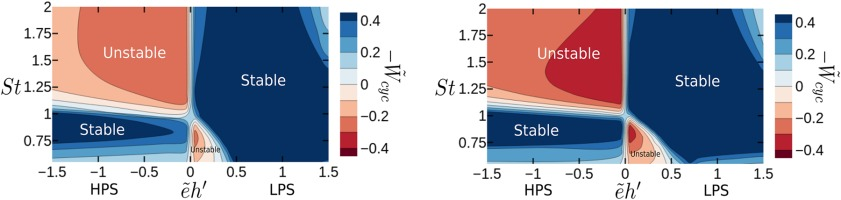### Steady state data derived from CFD non-linear analysis. The pressure ratio and the nondimensional frequency are kept constant, πT=1.5 and Ω~=3.35 respectively.

χsCd1Cd,2ηReh
0.230.970.930.8321.0003.32

To check quantitatively the accuracy of the analytical model comparison with a selected set of CFD results is included. Figure 8 displays the non-dimensional work-per-cycle as a function of the non-dimensional frequency ratio, St. The simulations are obtained by keeping constant the non-dimensional discharge time, Ω~=3.35, and the torsion center distance. As predicted by the model, for a given torsion center the resonant condition is always stable and is achieved close to St1. That condition corresponds to the maximum of the W~cyc (see Equation (7)). The matching is good for all the operating conditions including the cases where the torsion center is close to the seal (Figure 8b) and far away from the seal (Figure 8b). Both, the critical value of St and the asymptotic trend with dimensionless frequency are well predicted.

### Dimensionless work-per-cycle as a function of the frequency ratio, St. Comparison between the analytical model and the linearized Navier-Stokes simulations of a two fin straight seal operating at constant pressure ratio and frequency, πT=1.5 and Ω~=3.35, for selected torsion centers. (a) e~h′=−0.4(r/L=−4) (b) e~h′=0.05(r/L=0.5) (c) e~h′=0.7(r/L=7).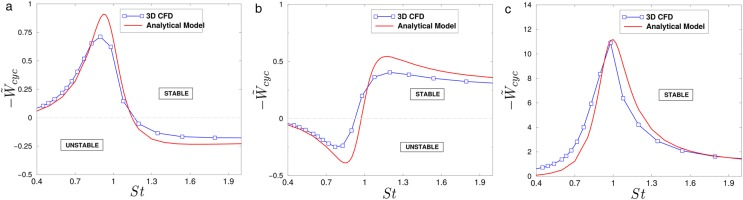## Concluding remarks

The aeroelastic stability of labyrinth seals under different working conditions and vibration mode-shapes has been compared with the predictions of an analytical model. It has been found that it is essential to inform the model with the correct parameters derived from the steady-state numerical simulations, especially the effective gaps and the amount of kinetic energy carried over to the downstream seal, which are the most critical parameters to allow a proper comparison with the model. The non-isentropic perturbations described in the high-order model have been observed in the CFD simulations at low vibration frequencies as expected. The largest discrepancies between the numerical results and the model predictions have been obtained when the discharge time of the seal is much smaller than the characteristic time of the vibration (Ω1), especially when the seal is operating at high-pressure ratios and the support is far from the seal. However, this regime is of modest engineering interest since in practice these frequencies are extremely low. In this context, the non-uniformity of the unsteady pressure field in the inter-fin cavity due to the influence of the outer cavities has been highlighted. The accuracy of the analytical model has been tested finally including the effect of the circumferential variations. The predictions of the analytical model have been compared with a large set of linear CFD simulations for a seal operating at intermediate pressure ratios. The matching with the analytical model is excellent in the whole range of the tested operating conditions. It is concluded that the model can predict not only the trends of the work-per-cycle of the seal but also quantitative results.

## Nomenclature

a0

Speed of sound in the cavity

CV

h

Seal pressure function

H

Fin clearance

HPS

High-Pressure Side

L

Seal cavity length

LPS

Low-Pressure Side

m˙

Mass flow rate

ND

Nodal diameter

pc

Cavity static pressure

pe

Exit static pressure

P0

Inlet total pressure

Pc0

Cavity effective total pressure

r

Torsion center position

R

s

Cavity height

St

Vibration-to-acoustic frequency ratio

CC

Cavity center

td

=pc,sVc.sm˙sa02. Discharge time

Wcyc

Work per cycle

s¯

=s/(γHh). Nondimensional height

Re

=m˙/(2πRμ) Reynolds number based on gap

m˙id

=P0iAiRTπi(γ+1)/2γ2γγ1(πi(γ1)/γ1) Ideal mass flow rate

### Greek symbols

γ

Heat capacity ratio

π

=P0/pc. Cavity pressure ratio

πT

=P0/pe. Total pressure ratio

πc

=πT/π. Pressure ratios relationship

ω

χ

=(Pc0pc)/(P0pc)/Kinetic carry-over coefficient

Ω

=ωtd Non-dimensional discharge time

Δθ

Rotation angle

τ

Non-dimensional time

### Super-scripts

~

Non-dimensional values

Time perturbation

e

Exit

c

Cavity

cyc

cycle

s